# A First Book on Quantum Field Theory

## Amitabha Lahiri, Palash B. Pal

### Errata and Addenda for the 1st edition

• To inform the authors of any error in the book that is not listed here, click here.

## Errata compiled so far

### Errors in mathematical formulas

Page Position Replace by Thanks go to
9 Ex. 1.4 anticommutation relation anticommutation relations [a,a]+=0 and R. N. Mohapatra, U of Maryland, College Park
12 Eq. (2.3) The last term, containing the partial time derivative, should be deleted since the variations of q are being taken at a given time. In the text following this equation, the sentence "We will assume .... vanishes" should be deleted as well. Shamik Gupta, Calcutta University
17 Ex. (2.3) The first term in the Lagrangian has both vector indices as subscripts. One of them should be a superscript. M. B. Einhorn, U of Michigan
41 Eq. (3.64) d4x d4 R. P. Malik, S N Bose Centre, Calcutta
43 Eq. (3.80) E Ep R. P. Malik, S N Bose Centre, Calcutta
57 Eq.(4.61)
and (4.62)

v+
v-

-v-
v+
to match with other texts (note).
M. B. Einhorn, U of Michigan
60 Ex. (4.16) Show that it is impossible to find a linear combination of the u-spinors of Eq. (4.60) that would be an eigenstate of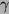5, unless the fermion is massless. Further, for a massless fermion, show that the eigenstates of5 are the helicity eigenstates.
63 Eq. (4.89)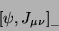63 Ex. (4.19) Add the following Hint to the problem:
You will have to use Eq. (4.101).

66 Eq. (4.108) d4x d4 R. P. Malik, S N Bose Centre, Calcutta
118 Ex. 7.4 with h substituted by |hʼ| with h2 substituted by
|hʼ|2 / (1 - 4m2/M2)
Gabriel Sanchez-Colon, CINVESTAV, Mexico
146 Eq. (8.21) M2 ½M2
149 Eqs. (8.32),
(8.33)
There should not be any minus sign on the right hand side. Sean Murray, National University of Ireland, Galway
154 After Eq. (8.55) Delete the text "Notice the negative .... sign-flipped commutator."
154 After Eq. (8.57) This is related to the problem of the sign-flipped commutator of A0 with Pi0 This is related to the wrong normalization of epsilon0 as shown in Eqs. (8.47) and (8.48)
157 Eq. (8.71) There should be a minus sign in front of the quantity on the right hand side. Sergio Gaudio, Boston College
238 Eq. 11.94 W1 = - ½ q2 (F1 + 2mp F2)2 W1 = ½ q2 (F1 - 2mp F2)2
300 Eq. (13.64), 2nd line In the denominator of the last term, the quantity 2k.k' has the wrong sign. It should be +2k.k' instead of -2k.k'. Rashidul Islam, S. N. Bose Center, Calcutta
314 Fig 14.1 In the Feynman rule for the quartic coupling, the overall sign in front of the second line should be negative.
325 Eq (15.5) The structure function factor in the rightmost term should be fbca instead of fabc. These two are equal for hermitian geneators, but not for the non-hermitian generators used here.
327 Eq. (15.23)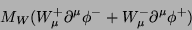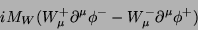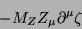C. S. Aulakh, Punjab University, Chandigarh
328 Eq. (15.24)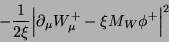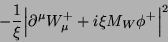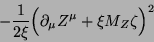333 Eq (15.47), 2nd line There should be a factor of   γ5 on the right side of the equation as well. William Kaufmann, Arizona State University, Tempe
341 after Eq. (15.79) The gAgV terms coming from here will contain only one trace ... added with the complex conjugate term, they will vanish The gAgV terms will vanish because they multiply one trace which is symmetric in the Lorentz indices and another which is antisymmetric,
357 Eq. (A.31) There should be a minus sign in front of the quantity on the right hand side. Monica Deep, Punjab University, Chandigarh

### Errors in other places

Page Where Replace by Thanks go to
3 Line 9 creation of annihilation of creation and annihilation of R. P. Malik, S N Bose Centre, Calcutta
44 Line 7 after Eq. (3.83) particle a time particle at time R. P. Malik, S N Bose Centre, Calcutta
53 Ex. 4.5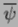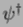0 (sincenot defined until Eq. 4.46 on page 54) S. Mukherji, Institute of Physics, Bhubaneshwar
54 Ex 4.7 Eq. (4.47), from the right by Eq. (4.47), by
85 last line particle particles R. P. Malik, S N Bose Centre, Calcutta
89 Line 10 the the points the points R. P. Malik, S N Bose Centre, Calcutta
115 Ex 7.2 more general when more general case when
195 7th line from bottom electro-electron scattering electron-electron scattering
205 1st line transforms that same way transforms the same way
253 5th line after Eq 12.31 a blob at both vertices blobs at both vertices
340 Line 6 the the electron the electron
364 Index entry for Dirac matrices, contraction formulas 173 174
Back cover: Box, Line 2  textbook at textbook for
Back cover: Box, Line 10  week weak